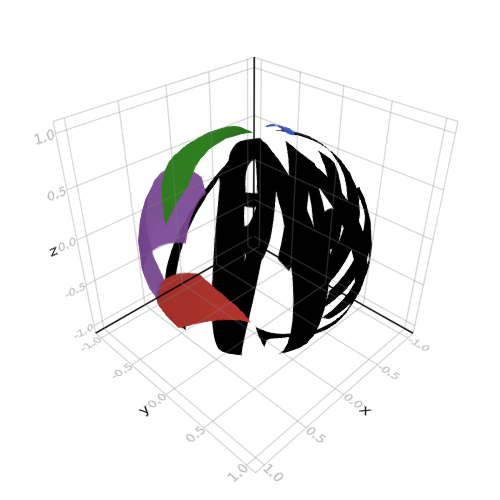## Image on Surface Sphere

```

using AbstractPlotting

n = 20
θ = [0;(0.5:n-0.5)/n;1]
φ = [(0:2n-2)*2/(2n-1);2]
x = [cospi(φ)*sinpi(θ) for θ in θ, φ in φ]
y = [sinpi(φ)*sinpi(θ) for θ in θ, φ in φ]
z = [cospi(θ) for θ in θ, φ in φ]
rand([-1f0, 1f0], 3)
pts = vec(Point3f0.(x, y, z))
surface(x, y, z, color = AbstractPlotting.logo(), transparency = true)

```